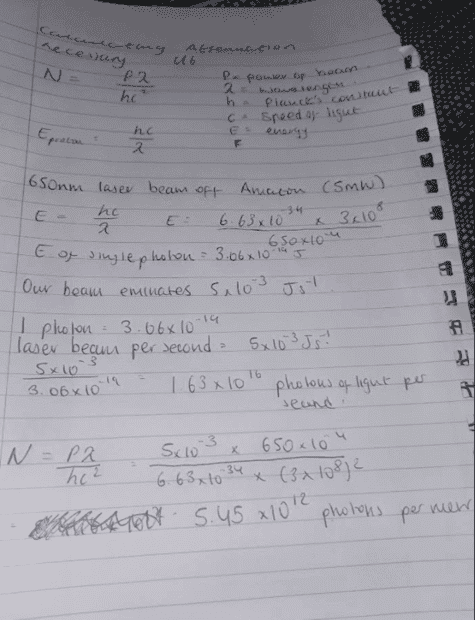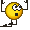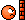# Calculating attenuation necessary to reduce number of photons

• kevincb672
Thanks for the help!In summary, the attenuation necessary to reduce the number of photons in a beam to single-photon levels is N approximately 1 or 2.f

#### kevincb672

Homework Statement
How are we to calculate the attenuation necessary to reduce the number of photons emitted from a laser beam to one photon per meter?
Relevant Equations
N = Plambda/hc(^2)
where N = photons per meter, P = power of beam, lambda = wave length, h = planck's constant, c = speed of light
E = hc/lambda
where E = energy of single photon
Hi there. I am attempting to do calculations for my own project, the question being what is the attenuation necessary to reduce the number of photons in a beam to single-photon levels. N approximately 1 or 2.

The laser in question is a 650nm 5mW laser.
I have solved the energy per photon 3.06*10(^-19) J.
I have solved the number of photons emitted from the laser beam per second 1.63*10(^16) photons of light per second.
I am struggling to find the photons per meter. I may have got the previous calculations wrong as well.
My calculations suggest 5.44662309×10(^12). A small part of me wants to be this wrong as this is if I am right entailing the need for the laser to be attenuated by 10x(^-12) so 12 orders of magnitude. If that is right of course.

I am very appreciative of any and all help. I've probably got this all wrong lol.

Thanks

I am struggling to find the photons per meter.
Imagine you shoot the beam into space for one second. How long is the beam in meters and how many photons are in it?

Last edited:
•kevincb672
Hello @kevincb672 ,##\qquad##!
My calculations suggest 5.44662309×10(^12).
N/c is 5.46 107 according to my calculations ...

##\ ##

Last edited:
•kevincb672
Imagine you shoot the beam into space for one second. How long is the beam in meters and how many photons are in it?
With a length to detector 2m is it correct to say the beam has 1.63398693×10(^16) photons? Apologies for my own ignorance.
Hello @kevincb672 ,##\qquad##!
My calculations suggest 5.44662309×10(^12).
N/c is 5.46 107 according to my calculations ...

##\ ##
Hi that does look right! Do you mind running through this a little bit, just so I can grasp it entirely, thanks again..

It's your own ##N = P\lambda/(hc^2)##

When I see ##\ 1.63\times 10^{16} ## divided by ##3\times 10^8\ ##, I expect something like ##5.5\times 10^7## !

Always estimate before grabbing a calculator.​

And if you have 1 digit accuracy data (5 mW), there is no point in presenting 9 digits results (not even intermediate).

Finally: 1 second of beam is ##3\times 10^8\ ## meters long. That's the distance over which the ##\ 1.63\times 10^{16} \ ## photons are spread out...

•kevincb672
With a length to detector 2m is it correct to say the beam has 1.63398693×10(^16) photons? Apologies for my own ignorance.
Show your work. I don't know how you came to this solution.

•kevincb672
Show your work. I don't know how you came to this solution.my wrong workings out but corrected now, your logic of "Imagine you shoot the beam into space for one second. How long is the beam in meters and how many photons are in it?" went straight over my head but I get it now!
It's your own ##N = P\lambda/(hc^2)##

When I see ##\ 1.63\times 10^{16} ## divided by ##3\times 10^8\ ##, I expect something like ##5.5\times 10^7## !

Always estimate before grabbing a calculator.​

And if you have 1 digit accuracy data (5 mW), there is no point in presenting 9 digits results (not even intermediate).

Finally: 1 second of beam is ##3\times 10^8\ ## meters long. That's the distance over which the ##\ 1.63\times 10^{16} \ ## photons are spread out...
Ah okay, I see! That helps me out. I can't understand why I didn't put two and two together realize that 1 second of the beam travels 3x10^8m and just put this under the number of photons. Always trying to improve my maths and common sensethanks sir•Drakkith
I still see a 5.45 1012 ?

How much is 650 nm in meters ?
Remember that nano means nine ...

##\ ##

•kevincb672
I still see a 5.45 1012 ?

How much is 650 nm in meters ?
Remember that nano means nine ...

##\ ##Correcting that silly error gets me the right answer as you had. I will refer myself back to primary school.

•BvU and berkeman